Version: 5.2.1

### 7Axis Transforms and Ticks

#### 7.1Axis Transforms

The x, y and z axes for any plot can be independently transformed by parameterizing the plot on different plot-x-transform, plot-y-transform and plot-z-transform values. For example, to plot the x axis with a log transform:
 > (parameterize ([plot-x-transform  log-transform]) (plot (function sin 1 100)))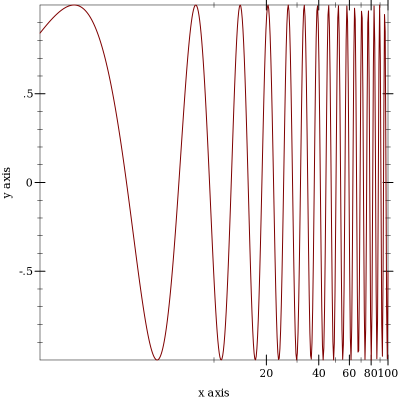Most log-transformed plots use different ticks than the default, uniformly spaced ticks, however. To put log ticks on the x axis, set the plot-x-ticks parameter:
 > (parameterize ([plot-x-transform  log-transform] [plot-x-ticks      (log-ticks)]) (plot (function sin 1 100)))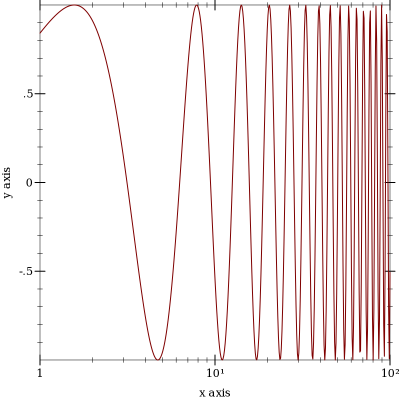See Axis Ticks for more details on parameterizing a plot’s axis ticks.

To sample nonlinearly, the inverse of a transform is applied to linearly sampled points. See make-axis-transform and nonlinear-seq. Renderers cooperate with the current transforms by sampling nonlinearly. For example,
 > (parameterize ([plot-x-transform  log-transform]) (plot3d (surface3d + 0.01 1 0.01 1)))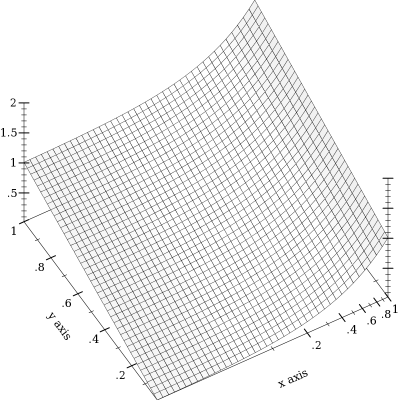Notice that the surface is sampled uniformly in appearance even though the x-axis ticks are not spaced uniformly.

Transforms are applied to the primitive shapes that comprise a plot:
 > (parameterize ([plot-x-transform  log-transform]) (plot3d (surface3d + 0.01 1 0.01 1 #:samples 3)))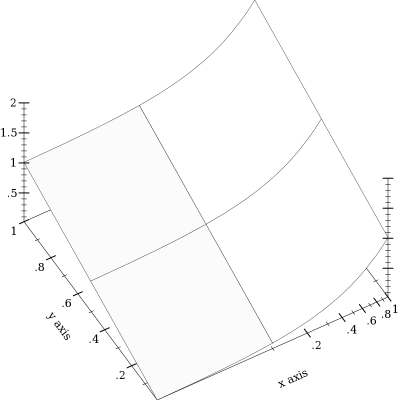Here, the renderer returned by surface3d does not have to bend the polygons it draws; plot3d does this automatically (by recursive subdivision).

 (plot-x-transform) → axis-transform/c (plot-x-transform transform) → void? transform : axis-transform/c
 = id-transform
 (plot-y-transform) → axis-transform/c (plot-y-transform transform) → void? transform : axis-transform/c
 = id-transform

 (plot-z-transform) → axis-transform/c (plot-z-transform transform) → void? transform : axis-transform/c
 = id-transform
Independent, per-axis, monotone, nonlinear transforms. PLoT comes with some typical (and some atypical) axis transforms, documented immediately below.

 id-transform : axis-transform/c
The identity axis transform, the default transform for all axes.

 log-transform : axis-transform/c
A log transform. Use this to generate plots with log-scale axes. Any such axis must have positive bounds.

The beginning of the Axis Transforms and Ticks section has a working example. An example of exceeding the bounds is
 > (parameterize ([plot-x-transform  log-transform]) (plot (function (λ (x) x) -1 1)))

log-transform: expects type <positive real> as 1st

argument, given: -1; other arguments were: 1

See axis-transform-bound and axis-transform-append for ways to get around an axis transform’s bounds limitations.

 (stretch-transform a b scale) → axis-transform/c a : real? b : real? scale : (>/c 0)
Returns an axis transform that stretches a finite interval.

The following example uses a stretch-transform to draw attention to the interval [-1,1] in an illustration of the limit of sin(x)/x as x approaches zero (a critical part of proving the derivative of sin(x)):
 > (parameterize ([plot-x-transform  (stretch-transform -1 1 20)] [plot-x-ticks  (ticks-add (plot-x-ticks) '(-1 1))]) (plot (list (y-axis -1 #:ticks? #f) (y-axis 1 #:ticks? #f) (function (λ (x) (/ (sin x) x)) -14 14 #:width 2 #:color 4 #:label "y = sin(x)/x") (point-label (vector 0 1) "y → 1 as x → 0" #:anchor 'bottom-right)) #:y-max 1.2))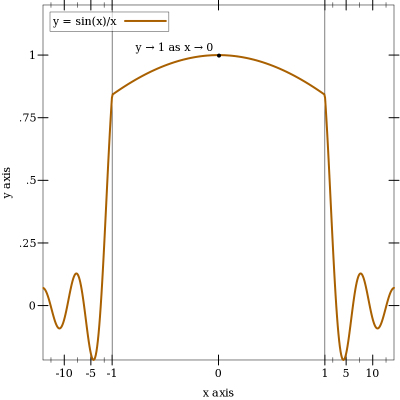(collapse-transform a b) → axis-transform/c a : real? b : real?
Returns an axis transform that collapses a finite interval to its midpoint. For example, to remove part of the long, boring asymptotic approach of atan(x) toward π/2:
 > (parameterize ([plot-x-transform  (collapse-transform 50 150)]) (plot (function atan 10 200 #:label "y = atan(x)") #:legend-anchor 'center))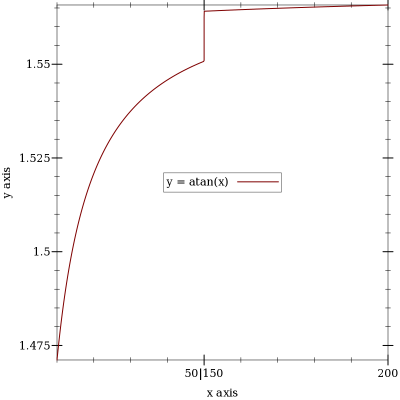In this case, there were already ticks at the collapsed interval’s endpoints. If there had not been, it would have been necessary to use ticks-add to let viewers know precisely the interval that was collapsed. (See stretch-transform for an example.)

 cbrt-transform : axis-transform/c
A “cube-root” transform, mostly used for testing. Unlike the log transform, it is defined on the entire real line, making it better for testing the appearance of plots with nonlinearly transformed axes.

 (hand-drawn-transform freq) → axis-transform/c freq : (>/c 0)
An extremely important test case, which makes sure that PLoT can use any monotone, invertible function as an axis transform. The freq parameter controls the “shakiness” of the transform. At high values, it makes plots look like Peanuts cartoons.

Examples:

 > (parameterize ([plot-x-transform  (hand-drawn-transform 200)] [plot-y-transform  (hand-drawn-transform 200)]) (plot (function sqr -1 1)))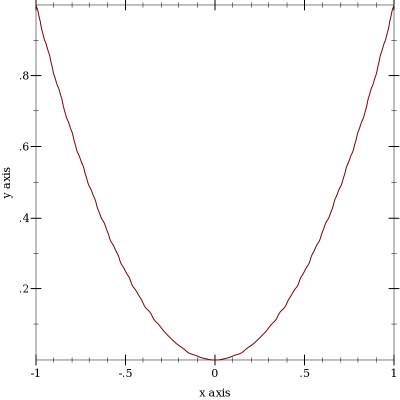> (parameterize ([plot-x-transform  (hand-drawn-transform 50)] [plot-y-transform  (hand-drawn-transform 50)] [plot-z-transform  (hand-drawn-transform 50)]) (plot3d (contour-intervals3d (λ (x y) (- (sqr x) (sqr y))) -1 1 -1 1 #:samples 9)))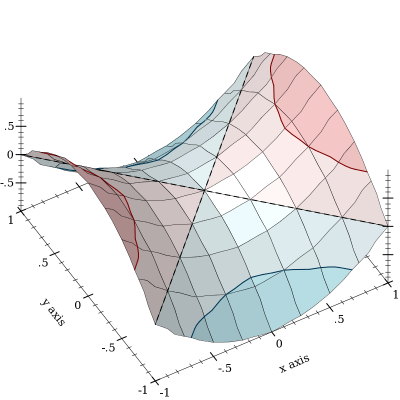axis-transform/c : contract?
 =
The contract for axis transforms.

The easiest ways to construct novel axis transforms are to use the axis transform combinators axis-transform-append, axis-transform-bound and axis-transform-compose, or to apply make-axis-transform to an invertible-function.

 (axis-transform-append t1 t2 mid) → axis-transform/c t1 : axis-transform/c t2 : axis-transform/c mid : real?
Returns an axis transform that transforms values less than mid like t1, and transforms values greater than mid like t2. (Whether it transforms mid like t1 or t2 is immaterial, as a transformed mid is equal to mid either way.)

Example:

 > (parameterize ([plot-x-transform  (axis-transform-append (stretch-transform -2 -1 10) (stretch-transform 1 2 10) 0)]) (plot (function (λ (x) x) -3 3)))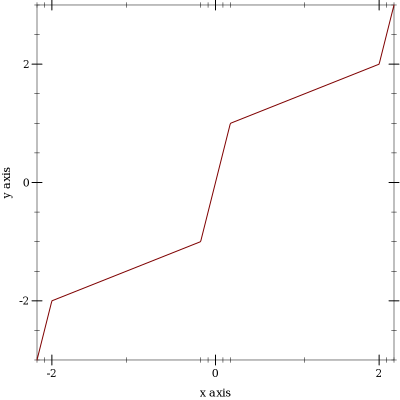(axis-transform-bound t a b) → axis-transform/c t : axis-transform/c a : real? b : real?

=

 (axis-transform-append (axis-transform-append id-transform t a) id-transform b)
Returns an axis transform that transforms values like t does in the interval [a,b], but like the identity transform outside of it. For example, to bound log-transform to an interval in which it is well-defined,
 > (parameterize ([plot-x-transform  (axis-transform-bound log-transform 0.01 +inf.0)]) (plot (function (λ (x) x) -4 8 #:label "y = x")))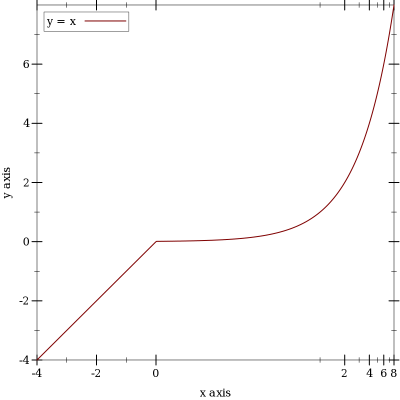(axis-transform-compose t1 t2) → axis-transform/c t1 : axis-transform/c t2 : axis-transform/c
Composes two axis transforms. For example, to collapse part of a log-transformed axis, try something like
 > (parameterize ([plot-x-transform  (axis-transform-compose log-transform (collapse-transform 2 4))]) (plot (function (λ (x) x) 1 5)))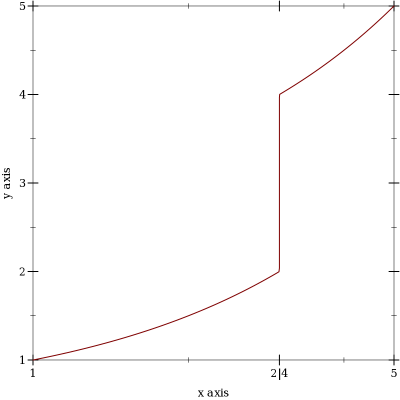Argument order matters, but predicting the effects of exchanging arguments can be difficult. Fortunately, the effects are usually slight.

 (make-axis-transform fun) → axis-transform/c fun : invertible-function?
Given a monotone invertible-function, returns an axis transform. Monotonicity is necessary, but cannot be enforced. The inverse is used to take samples uniformly along transformed axes (see nonlinear-seq).

Example:

 > (parameterize ([plot-y-transform  (make-axis-transform (invertible-function sqrt sqr))]) (plot (function (λ (x) x) 0 5)))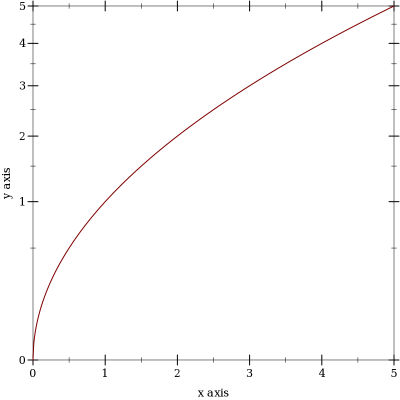An axis transform created by make-axis-transform (or by any of the above combinators) does not transform the endpoints of an axis’s bounds, to within floating-point error. For example,
 > (match-let ([(invertible-function f g) (apply-axis-transform log-transform 1 3)]) (define xs '(1 2 3)) (define new-xs (map f xs)) (define old-xs (map g new-xs)) (values new-xs old-xs))
 '(1.0 2.2618595071429146 3.0) '(1.0 1.9999999999999998 3.0000000000000004)

Technically, fun does not need to be truly invertible. Given fun = (invertible-function f g), it is enough for f to be a left inverse of g; that is, always (f (g x)) = x but not necessarily (g (f x)) = x. If f and g had to be strict inverses of each other, there could be no collapse-transform.

 (apply-axis-transform t x-min x-max) → invertible-function? t : axis-transform/c x-min : real? x-max : real?
 = (t x-min x-max id-function)
Returns an invertible function that transforms axis points within the given axis bounds. This convenience function is used internally to transform points before rendering, but is provided for completeness.

#### 7.2Axis Ticks

Each plot axis has two indepedent sets of ticks: the near ticks and the far ticks.

 (plot-x-ticks) → ticks? (plot-x-ticks ticks) → void? ticks : ticks?
 =
 (plot-x-far-ticks) → ticks? (plot-x-far-ticks ticks) → void? ticks : ticks?
 =
 (plot-y-ticks) → ticks? (plot-y-ticks ticks) → void? ticks : ticks?
 =
 (plot-y-far-ticks) → ticks? (plot-y-far-ticks ticks) → void? ticks : ticks?
 =
 (plot-z-ticks) → ticks? (plot-z-ticks ticks) → void? ticks : ticks?
 =

 (plot-z-far-ticks) → ticks? (plot-z-far-ticks ticks) → void? ticks : ticks?
 =

Example:

 > (parameterize ([plot-x-label      "Near x axis"] [plot-y-label      "Near y axis"] [plot-z-label      "Near z axis"] [plot-x-ticks      (date-ticks)] [plot-y-ticks      (time-ticks)] [plot-z-ticks      (fraction-ticks)] [plot-x-far-label  "Far x axis"] [plot-y-far-label  "Far y axis"] [plot-z-far-label  "Far z axis"] [plot-x-far-ticks  (linear-ticks)] [plot-y-far-ticks  (currency-ticks)] [plot-z-far-ticks  (log-ticks #:base 2)]) (plot3d (lines3d '(#(1 1 1) #(40000000 4 4)) #:style 'transparent) #:angle 45 #:altitude 50 #:title "Axis Names and Tick Locations"))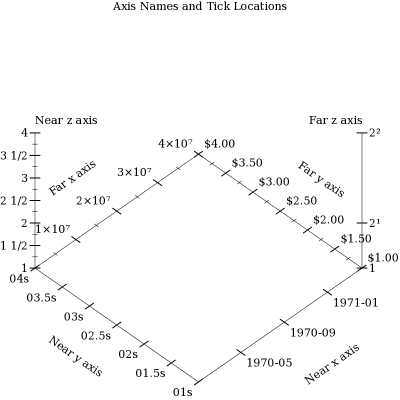At any #:angle, the far x and y ticks are behind the plot, and the far z ticks are on the right. Far ticks are drawn, but not labeled, if they are identical to their corresponding near ticks. They are always identical by default.

Major ticks are longer than minor ticks. Major tick labels are always drawn unless collapsed with a nearby tick. Minor tick labels are never drawn.

Renderers produced by contours and contour-intervals use the value of plot-z-ticks to place and label contour lines. For example, compare plots of the same function renderered using both contour-intervals and contour-intervals3d:
 > (parameterize ([plot-z-ticks  (currency-ticks)]) (define (saddle x y) (- (sqr x) (sqr y))) (values (plot (contour-intervals saddle -1 1 -1 1 #:label "z") #:legend-anchor 'center) (plot3d (contour-intervals3d saddle -1 1 -1 1 #:label "z") #:legend-anchor 'center)))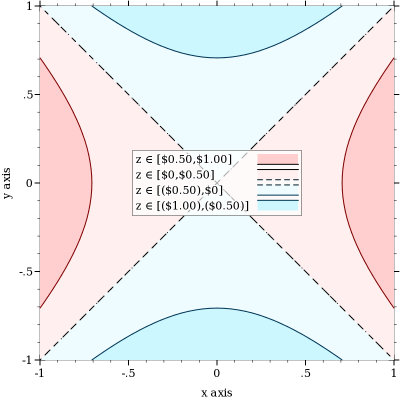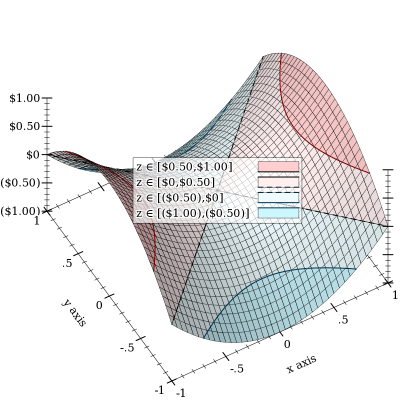(contour-ticks z-ticks z-min z-max levels intervals?) → (listof tick?)
z-ticks : ticks?
z-min : real?
z-max : real?
levels : (or/c 'auto exact-positive-integer? (listof real?))
intervals? : boolean?
Returns the ticks used for contour values. This is used internally by renderers returned from contours, contour-intervals, contours3d, contour-intervals3d, and isosurfaces3d, but is provided for completeness.

When levels is 'auto, the returned values do not correspond exactly with the values of ticks returned by z-ticks: they might be missing the endpoint values. For example,
 > (map pre-tick-value (filter pre-tick-major? ((plot-z-ticks) 0 1)))

'(0 1/5 2/5 3/5 4/5 1)

 > (map pre-tick-value (contour-ticks (plot-z-ticks) 0 1 'auto #f))

'(1/5 2/5 3/5 4/5)

 (plot-d-ticks) → ticks? (plot-d-ticks ticks) → void? ticks : ticks?
 =
The ticks used for default isosurface values in isosurfaces3d.

 (plot-r-ticks) → ticks? (plot-r-ticks ticks) → void? ticks : ticks?
 =
The ticks used for radius lines in polar-axes.

 (struct ticks (layout format) #:extra-constructor-name make-ticks)
layout : ticks-layout/c
format : ticks-format/c
A ticks for a near or far axis consists of a layout function, which determines the number of ticks and where they will be placed, and a format function, which determines the ticks’ labels.

 (ticks-default-number) → exact-positive-integer? (ticks-default-number number) → void? number : exact-positive-integer?
 = 4
Most tick layout functions (and thus their corresponding ticks-constructing functions) have a #:number keyword argument with default (ticks-default-number). What the number means depends on the tick layout function. Most use it for an average number of major ticks.

It is unlikely to mean the exact number of major ticks. Without adjusting the number of ticks, layout functions usually cannot find uniformly spaced ticks that will have simple labels after formatting. For example, the following plot shows the actual number of major ticks for the interval [0,x] when the requested number of ticks is 8, as generated by linear-ticks-layout:
 > (plot (function (λ (x) (count pre-tick-major? ((linear-ticks-layout #:number 8) 0 x))) 0.1 10) #:x-label "Interval [0,x]" #:y-label "Number of ticks")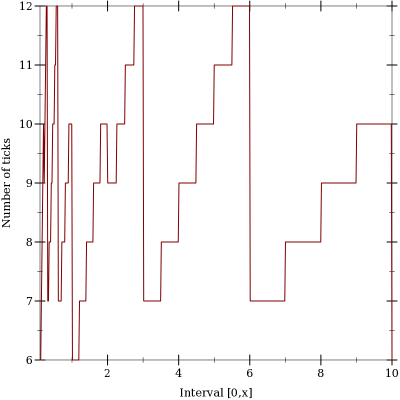##### 7.2.1Linear Ticks

 (linear-ticks-layout [ #:number number #:base base #:divisors divisors]) → ticks-layout/c
number : exact-positive-integer? = (ticks-default-number)
base : (and/c exact-integer? (>=/c 2)) = 10
divisors : (listof exact-positive-integer?) = '(1 2 4 5)
 (linear-ticks-format) → ticks-format/c

 (linear-ticks [ #:number number #:base base #:divisors divisors]) → ticks?
number : exact-positive-integer? = (ticks-default-number)
base : (and/c exact-integer? (>=/c 2)) = 10
divisors : (listof exact-positive-integer?) = '(1 2 4 5)

=

 (ticks (linear-ticks-layout #:number number #:base base #:divisors divisors) (linear-ticks-format))
The layout function, format function, and combined ticks for uniformly spaced ticks.

To lay out ticks, linear-ticks-layout finds the power of base closest to the axis interval size, chooses a simple first tick, and then chooses a skip length using divisors that maximizes the number of ticks without exceeding number. For strategic use of non-default arguments, see bit/byte-ticks, currency-ticks, and fraction-ticks. The default arguments correspond to the standard 1-2-5-in-base-10 rule used almost everywhere in plot tick layout.

To format ticks, linear-ticks-format uses real->plot-label, and uses digits-for-range to determine the maximum number of fractional digits in the decimal expansion.

##### 7.2.2Log Ticks

 (log-ticks-layout [ #:number number #:base base]) → ticks-layout/c
number : exact-positive-integer? = (ticks-default-number)
base : (and/c exact-integer? (>=/c 2)) = 10
 (log-ticks-format [#:base base]) → ticks-format/c base : (and/c exact-integer? (>=/c 2)) = 10

 (log-ticks [#:number number #:base base]) → ticks? number : exact-positive-integer? = (ticks-default-number) base : (and/c exact-integer? (>=/c 2)) = 10

=

 (ticks (log-ticks-layout #:number number #:base base) (log-ticks-format #:base base))
The layout function, format function, and combined ticks for exponentially spaced major ticks. (The minor ticks between are uniformly spaced.) Use these ticks for log-transformed axes, because when exponentially spaced tick positions are log-transformed, they become uniformly spaced.

The #:base keyword argument is the logarithm base. See plot-z-far-ticks for an example of use.

##### 7.2.3Date Ticks

 (date-ticks-layout [#:number number]) → ticks-layout/c number : exact-positive-integer? = (ticks-default-number)
 (date-ticks-format [#:formats formats]) → ticks-format/c formats : (listof string?) = (date-ticks-formats)

 (date-ticks [ #:number number #:formats formats]) → ticks?
number : exact-positive-integer? = (ticks-default-number)
formats : (listof string?) = (date-ticks-formats)

=

 (ticks (date-ticks-layout #:number number) (date-ticks-format #:formats formats))
The layout function, format function, and combined ticks for uniformly spaced ticks with date labels.

These axis ticks regard values as being in seconds since a system-dependent Universal Coordinated Time (UTC) epoch. (For example, the Unix and Mac OS X epoch is January 1, 1970 UTC, and the Windows epoch is January 1, 1601 UTC.) Use date->seconds to convert local dates to seconds, or datetime->real to convert dates to UTC seconds in a way that accounts for time zone offsets.

Actually, date-ticks-layout does not always space ticks quite uniformly. For example, it rounds ticks that are spaced about one month apart or more to the nearest month. Generally, date-ticks-layout tries to place ticks at minute, hour, day, week, month and year boundaries, as well as common multiples such as 90 days or 6 months.

To try to avoid displaying overlapping labels, date-ticks-format chooses date formats from formats for which labels will contain no redundant information.

All the format specifiers given in srfi/19 (which are derived from Unix’s date command), except those that represent time zones, are allowed in date format strings.

 (date-ticks-formats) → (listof string?) (date-ticks-formats formats) → void? formats : (listof string?)
 = 24h-descending-date-ticks-formats
The default date formats.

 24h-descending-date-ticks-formats : (listof string?)

=

 '("~Y-~m-~d ~H:~M:~f" "~Y-~m-~d ~H:~M" "~Y-~m-~d ~Hh" "~Y-~m-~d" "~Y-~m" "~Y" "~m-~d ~H:~M:~f" "~m-~d ~H:~M" "~m-~d ~Hh" "~m-~d" "~H:~M:~f" "~H:~M" "~Hh" "~M:~fs" "~Mm" "~fs")
 12h-descending-date-ticks-formats : (listof string?)

=

 '("~Y-~m-~d ~I:~M:~f ~p" "~Y-~m-~d ~I:~M ~p" "~Y-~m-~d ~I ~p" "~Y-~m-~d" "~Y-~m" "~Y" "~m-~d ~I:~M:~f ~p" "~m-~d ~I:~M ~p" "~m-~d ~I ~p" "~m-~d" "~I:~M:~f ~p" "~I:~M ~p" "~I ~p" "~M:~fs" "~Mm" "~fs")

##### 7.2.4Time Ticks

 (time-ticks-layout [#:number number]) → ticks-layout/c number : exact-positive-integer? = (ticks-default-number)
 (time-ticks-format [#:formats formats]) → ticks-format/c formats : (listof string?) = (time-ticks-formats)

 (time-ticks [ #:number number #:formats formats]) → ticks?
number : exact-positive-integer? = (ticks-default-number)
formats : (listof string?) = (time-ticks-formats)

=

 (ticks (time-ticks-layout #:number number) (time-ticks-format #:formats formats))
The layout function, format function, and combined ticks for uniformly spaced ticks with time labels.

These axis ticks regard values as being in seconds. Use datetime->real to convert sql-time or plot-time values to seconds.

Generally, time-ticks-layout tries to place ticks at minute, hour and day boundaries, as well as common multiples such as 12 hours or 30 days.

To try to avoid displaying overlapping labels, time-ticks-format chooses a date format from formats for which labels will contain no redundant information.

All the time-related format specifiers given in srfi/19 (which are derived from Unix’s date command) are allowed in time format strings.

 (time-ticks-formats) → (listof string?) (time-ticks-formats formats) → void? formats : (listof string?)
 = 24h-descending-time-ticks-formats
The default time formats.

 24h-descending-time-ticks-formats : (listof string?)

=

 '("~dd ~H:~M:~f" "~dd ~H:~M" "~dd ~Hh" "~dd" "~H:~M:~f" "~H:~M" "~Hh" "~M:~fs" "~Mm" "~fs")
 12h-descending-time-ticks-formats : (listof string?)

=

 '("~dd ~I:~M:~f ~p" "~dd ~I:~M ~p" "~dd ~I ~p" "~dd" "~I:~M:~f ~p" "~I:~M ~p" "~I ~p" "~M:~fs" "~Mm" "~fs")

##### 7.2.5Currency Ticks

 (currency-ticks-format [ #:kind kind #:scales scales #:formats formats]) → ticks-format/c
kind : (or/c string? symbol?) = 'USD
scales : (listof string?) = (currency-ticks-scales)
 formats : (list/c string? string? string?) = (currency-ticks-formats)

 (currency-ticks [ #:number number #:kind kind #:scales scales #:formats formats]) → ticks?
number : exact-positive-integer? = (ticks-default-number)
kind : (or/c string? symbol?) = 'USD
scales : (listof string?) = (currency-ticks-scales)
 formats : (list/c string? string? string?) = (currency-ticks-formats)

=

 (ticks (linear-ticks-layout #:number number) (currency-ticks-format #:kind kind #:scales scales #:formats formats))
The format function and combined ticks for uniformly spaced ticks with currency labels.

The #:kind keyword argument is either a string containing the currency symbol, or a currency code such as 'USD, 'GBP or 'EUR. The currency-ticks-format function can map most ISO 4217 currency codes to their corresponding currency symbol.

The #:scales keyword argument is a list of suffixes for each 103 scale, such as "K" (US thousand, or kilo), "bn" (UK short-scale billion) or "Md" (EU long-scale milliard). Off-scale amounts are given power-of-ten suffixes such as “×1021.”

The #:formats keyword argument is a list of three format strings, representing the formats of positive, negative, and zero amounts, respectively. The format specifiers are:
• "~\$": replaced by the currency symbol

• "~w": replaced by the whole part of the amount

• "~f": replaced by the fractional part, with 2 or more decimal digits

• "~s": replaced by the scale suffix

• "~~": replaced by “~”

 (currency-ticks-scales) → (listof string?) (currency-ticks-scales scales) → void? scales : (listof string?)
 = us-currency-scales

 (currency-ticks-formats) → (list/c string? string? string?) (currency-ticks-formats formats) → void? formats : (list/c string? string? string?)
 = us-currency-formats
The default currency scales and formats.

For example, a PLoT user in France would probably begin programs with
 (require plot) (currency-ticks-scales eu-currency-scales) (currency-ticks-formats eu-currency-formats)
and use (currency-ticks #:kind 'EUR) for local currency or (currency-ticks #:kind 'JPY) for Japanese Yen.

Cultural sensitivity notwithstanding, when writing for a local audience, it is generally considered proper to use local currency scales and formats for foreign currencies, but use the foreign currency symbol.

 us-currency-scales : (listof string?)
 = '("" "K" "M" "B" "T")
Short-scale suffix abbreviations as commonly used in the United States, Canada, and some other English-speaking countries. These stand for “kilo,” “million,” “billion,” and “trillion.”

 uk-currency-scales : (listof string?)
 = '("" "k" "m" "bn" "tr")
Short-scale suffix abbreviations as commonly used in the United Kingdom since switching to the short scale in 1974, and as currently recommended by the Daily Telegraph and Times style guides.

 eu-currency-scales : (listof string?)
 = '("" "K" "M" "Md" "B")
European Union long-scale suffix abbreviations, which stand for “kilo,” “million,” “milliard,” and “billion.”

The abbreviations actually used vary with geography, even within countries, but these seem to be common. Further long-scale suffix abbreviations such as for “billiard” are ommitted due to lack of even weak consensus.

 us-currency-formats : (list/c string? string? string?)
 = '("~\$~w.~f~s" "(~\$~w.~f~s)" "~\$0")
Common currency formats used in the United States.

 uk-currency-formats : (list/c string? string? string?)
 = '("~\$~w.~f~s" "-~\$~w.~f~s" "~\$0")
Common currency formats used in the United Kingdom. Note that it sensibly uses a negative sign to denote negative amounts.

 eu-currency-formats : (list/c string? string? string?)
 = '("~w,~f ~s~\$" "-~w,~f ~s~\$" "0 ~\$")
A guess at common currency formats for the European Union. Like scale suffixes, actual formats vary with geography, but currency formats can even vary with audience or tone.

##### 7.2.6Other Ticks

 no-ticks-layout : ticks-layout/c
 = (λ (x-min x-max) empty)
 no-ticks-format : ticks-format/c

=

 (λ (x-min x-max pre-ticks) (map (λ (_) "") pre-ticks))

 no-ticks : ticks?
 =
The layout function, format function, and combined ticks for no ticks whatsoever.

Example:

 > (parameterize ([plot-x-ticks  no-ticks] [plot-y-ticks  no-ticks] [plot-x-label  #f] [plot-y-label  #f]) (plot (list (polar-axes) (polar (λ (θ) 1/3)))))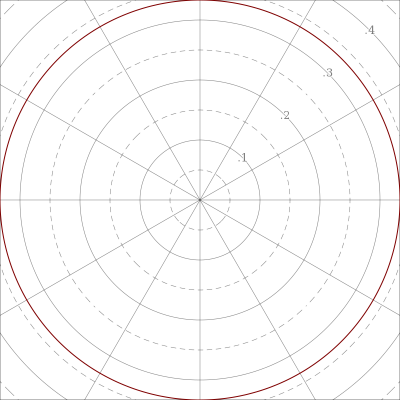(bit/byte-ticks-format [ #:size size #:kind kind]) → ticks-format/c
size : (or/c 'byte 'bit) = 'byte
kind : (or/c 'CS 'SI) = 'CS

 (bit/byte-ticks [ #:number number #:size size #:kind kind]) → ticks?
number : exact-positive-integer? = (ticks-default-number)
size : (or/c 'byte 'bit) = 'byte
kind : (or/c 'CS 'SI) = 'CS

=

 (define si? (eq? kind 'SI)) (ticks (linear-ticks-layout #:number number #:base (if si? 10 2) #:divisors (if si? '(1 2 4 5) '(1 2))) (bit/byte-ticks-format #:size size #:kind kind))
The format function and and combined ticks for bit or byte values.

The #:kind keyword argument indicates either International System of Units ('SI) suffixes, as used to communicate hard drive capacities, or Computer Science ('CS) suffixes, as used to communicate memory capacities.

 (fraction-ticks-format [ #:base base #:divisors divisors]) → ticks-format/c
base : (and/c exact-integer? (>=/c 2)) = 10
divisors : (listof exact-positive-integer?) = '(1 2 3 4 5)

 (fraction-ticks [ #:base base #:divisors divisors]) → ticks?
base : (and/c exact-integer? (>=/c 2)) = 10
divisors : (listof exact-positive-integer?) = '(1 2 3 4 5)

=

 (ticks (linear-ticks #:base base #:divisors divisors) (fraction-ticks-format #:base base #:divisors divisors))
The format function and and combined ticks for fraction-formatted values.

##### 7.2.7Tick Combinators

 (ticks-mimic thunk) → ticks? thunk : (-> ticks?)
Returns a ticks that mimics the given ticks returned by thunk. Used in default values for plot-x-far-ticks, plot-y-far-ticks and plot-z-far-ticks to ensure that, unless one of these parameters is changed, the far tick labels are not drawn.

 (ticks-add t xs [major?]) → ticks? t : ticks? xs : (listof real?) major? : boolean? = #t
Returns a new ticks that acts like t, except that it puts additional ticks at positions xs. If major? is true, the ticks at positions xs are all major ticks; otherwise, they are minor ticks.

 (ticks-scale t fun) → ticks? t : ticks? fun : invertible-function?
Returns a new ticks that acts like t, but for an axis transformed by fun. Unlike with typical Axis Transforms, fun is allowed to transform axis endpoints. (See make-axis-transform for an explanation about transforming endpoints.)

Use ticks-scale to plot values at multiple scales simultaneously, with one scale on the near axis and one scale on the far axis. The following example plots degrees Celsius on the left and degrees Farenheit on the right:
 > (parameterize ([plot-x-ticks      (time-ticks)] [plot-y-far-ticks  (ticks-scale (plot-y-ticks) (linear-scale 9/5 32))] [plot-y-label      "Temperature (°C)"] [plot-y-far-label  "Temperature (°F)"]) (define data (list #(0 0) #(15 0.6) #(30 9.5) #(45 10.0) #(60 16.6) #(75 41.6) #(90 42.7) #(105 65.5) #(120 78.9) #(135 78.9) #(150 131.1) #(165 151.1) #(180 176.2))) (plot (list (function (λ (x) (/ (sqr x) 180)) 0 180 #:style 'long-dash #:color 3 #:label "Trend") (lines data #:color 2 #:width 2) (points data #:color 1 #:line-width 2 #:label "Measured")) #:y-min -25 #:x-label "Time"))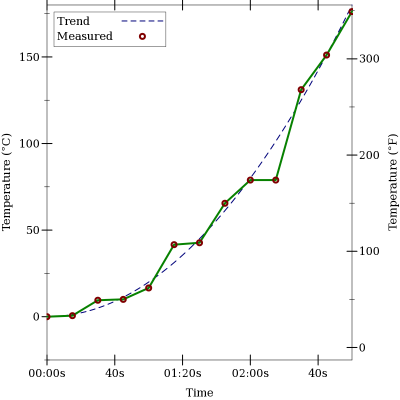##### 7.2.8Tick Data Types and Contracts

 (struct pre-tick (value major?) #:extra-constructor-name make-pre-tick)
value : real?
major? : boolean?
Represents a tick that has not yet been labeled.
 (struct tick pre-tick (label) #:extra-constructor-name make-tick)
label : string?
Represents a tick with a label.

 ticks-layout/c : contract?
 =
The contract for tick layout functions. Note that a layout function returns pre-ticks, or unlabeled ticks.

 ticks-format/c : contract?
 =
The contract for tick format functions. A format function receives axis bounds so it can determine how many decimal digits to display (usually by applying digits-for-range to the bounds).

#### 7.3Invertible Functions

 (struct invertible-function (f g) #:extra-constructor-name make-invertible-function)
f : (real? . -> . real?)
g : (real? . -> . real?)
Represents an invertible function. Used for Axis Transforms and by ticks-scale.

The function itself is f, and its inverse is g. Because real?s can be inexact, this invariant must be approximate and therefore cannot be enforced. (For example, (exp (log 10)) = 10.000000000000002.) The obligation to maintain it rests on whomever constructs one.

 id-function : invertible-function?
The identity function as an invertible-function.

 (invertible-compose f1 f2) → invertible-function? f1 : invertible-function? f2 : invertible-function?
Returns the composition of two invertible functions.

 (invertible-inverse h) → invertible-function? h : invertible-function?

=

 (match-define (invertible-function f g) h) (invertible-function g f)
Returns the inverse of an invertible function.

 (linear-scale m [b]) → invertible-function? m : rational? b : rational? = 0

=

 (invertible-function (λ (x) (+ (* m x) b)) (λ (y) (/ (- y b) m)))
Returns a one-dimensional linear scaling function, as an invertible-function. This function constructs the most common arguments to ticks-scale.